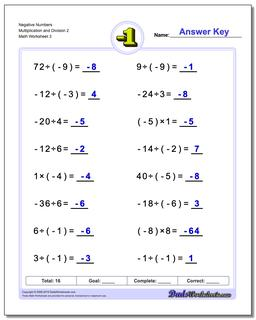PLEASE GO BACK AND USE THE BIG BLUE 'PRINT' BUTTON ON THE PAGE TO PRINT THE WORKSHEET CORRECTLY!Sorry for the trouble! The browser won't print the embedded worksheet PDF directly using the normal 'Print' command in the file menu, so you need to click the big 'Print' button to send just the worksheet and not the surrounding page to the printer.

# Math Worksheets: Negative Numbers: Negative Numbers: Negative Numbers Multiplication and Division 2 (Third Worksheet)## Negative Numbers Multiplication and Division 2 (Third Worksheet)

PropertyValue
DescriptionNegative Numbers Multiplication and Division 2: The worksheets in this section introduce negative numbers integers in multiplication and division math problems. All problems deal with smaller integers that can be solved without multi-digit multiplication or long division. (Third Worksheet)
Resource TypeWorksheet